Name:    Quiz 7.5-7.6

Multiple Choice
Identify the choice that best completes the statement or answers the question.

Find the volume.

1.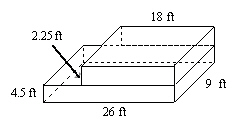a. 1053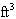c. 59.75b. 1579.5d. 1417.52.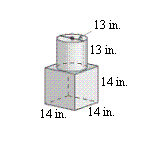a. 68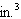c. 5,292b. 2,744 +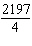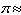4,468.6d. 2,744 + 2,1979,642.63.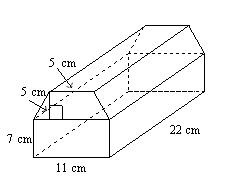a. 50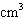c. 2,244b. 2,904d. 2,574The solids are similar.  Find the missing dimension(s).

4.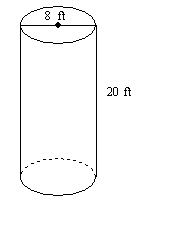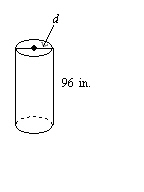a. 20 in. c. 38.4 ft b. 240 in. d. 38.4 in.

1.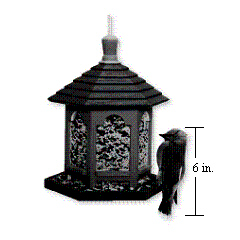A bird feeder is designed to feed birds who are at most 6 inches tall from head to tail. The hexagonal part of the bird feeder that holds the bird seed has a base area of 38 square inches. Estimate how much bird seed the bird feeder will hold. Explain how you found your estimate.

2.

A foam kick board used for swimming has two identical hand grips.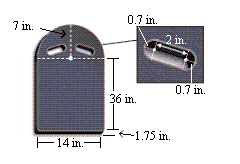a.   Find the volume of the kick board.
b.   One cubic inch of foam weighs about 0.007 pounds. How much does the kick board weigh?

Determine whether the solids are similar.

3.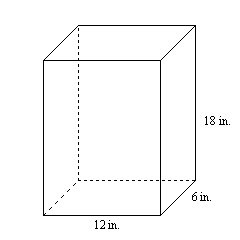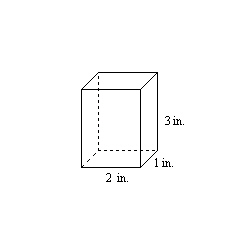The solids are similar. Find the surface area S and volume V of the smaller solid. Round your answers to the nearest tenth.

4.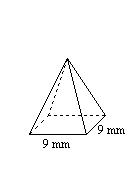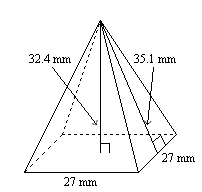5.

You have a small marble statue of Wolfgang Mozart that is 5 inches tall and weighs 8 pounds. The original statue in Vienna is 7 feet tall.
a.   Estimate the weight of the original statue. Explain your reasoning.
b.   If the original statue were 25 feet tall, how much would it weigh?